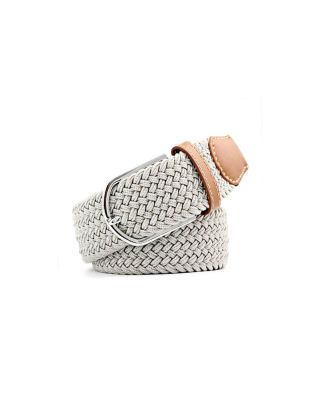# Wearables

View as Grid List

6 Items

Set Descending Direction
1.Golfoy Basics
Braided Stretchable Canvas Belt
Array (  => 7490  => 19118  => 19119  => 14036  => 19044  => 6784  => 8656  => 13724  => 14037  => 19122  => 8660  => 19121  => 19123  => 13725  => 19117  => 19036  => 19035  => 19115  => 19114  => 19120  => 19116  => 21371  => 22008  => 22009  => 22010  => 22016  => 22017  => 22018  => 22019  => 22020  => 22021  => 22022  => 22023  => 22024  => 22026  => 22027  => 22028 )
MRP. ₹ 999
Deal Price ₹ 595
You save: ₹ 404 (40% OFF)
Rating:
88%
View as Grid List

6 Items

Set Descending Direction